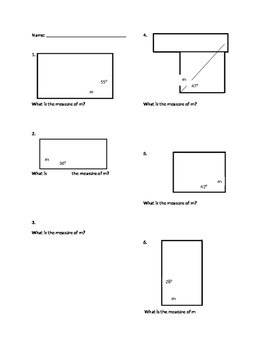# Finding a Missing Angle in a RectangleSubject
Resource Type
File Type
Word Document File (38 KB|1 page)
Standards
\$0.95
• Product Description
• Standards
6 problems of finding a missing angle in a rectangle.

The right angles of a rectangle are decomposed into two smaller angles. One smaller angle measure is given and students must find the missing one.
Recognize angle measure as additive. When an angle is decomposed into non-overlapping parts, the angle measure of the whole is the sum of the angle measures of the parts. Solve addition and subtraction problems to find unknown angles on a diagram in real world and mathematical problems, e.g., by using an equation with a symbol for the unknown angle measure.
Total Pages
1 page